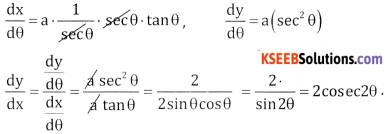# 2nd PUC Basic Maths Question Bank Chapter 18 Differential Calculus Ex 18.6

Students can Download Basic Maths Exercise 18.6 Questions and Answers, Notes Pdf, 2nd PUC Basic Maths Question Bank with Answers helps you to revise the complete Karnataka State Board Syllabus and score more marks in your examinations.

## Karnataka 2nd PUC Basic Maths Question Bank Chapter 18 Differential Calculus Ex 18.6

Part – A

2nd PUC Basic Maths Differential Calculus Ex 18.6 Two or Three Marks Questions and Answers

Question 1.
Find $$\frac{d y}{d x}$$ if
(a) x = $$a\left(t-\frac{1}{t}\right), y=a\left(t+\frac{1}{t}\right) 1$$
(b) x = e2t , y = log(2t + 1)
(c) x = log(1 + t), y = $$\frac{1}{1+t}$$
(d) x = log t, y = $$\frac{1}{t}$$
(e) x = 4t , y = $$\frac{4}{t}$$
(f) x = a sec θ, y = b tan θ.
(g) x = a(θ – sin θ), y = a(1 – cos θ)
(h) x = a cos(log t), y = a log(cos t)
(a) Given x = $$a\left(t-\frac{1}{t}\right), y=a\left(t+\frac{1}{t}\right) 1$$
Differentiate both w.r.t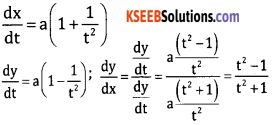(b) Given x = e2t , y = log(2t + 1)
Differentiate both w.r.t we get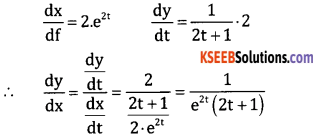(c) Given x = log (1+t), y = $$\frac{1}{1+t}$$
Differentiate both w.r.t x t, we get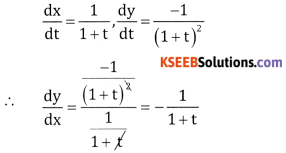(d) Given x = log t, y = $$\frac{1}{t}$$
Differentiate both w.r.t t, we get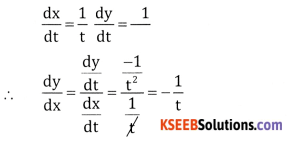(e) Given x = 4t y = $$\frac{4}{t}$$
Differentiate both w.r.t t, we get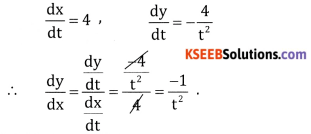(f) Given a = secθ, y = b tan θ
Differentiate both w.r.t. θ we get(g) Given x = a (θ – sin θ), y = a(1 – cosθ)
Differentiate both w.r.t θ(h) Given x = a cos(logt) y = a log(cos t)
Differentiate both w.r.t t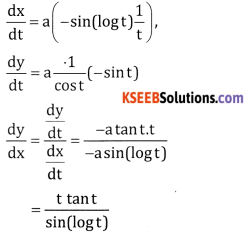Question 2.
Differentiate tan2 w.r.t cos2x.
Let u = tan2x, v = cos2x
Differentiate both w.r.t x
$$\frac{d u}{d x}$$ = 2tan x. sec2x,
$$\frac{d v}{d x}$$ = 2 cosx(-sinx)
∴ $$\frac{d u}{d v}$$ = $$\frac{\frac{d u}{d x}}{\frac{d v}{d x}}=\frac{2 \tan x \cdot \sec ^{2} x}{2 \sin x \cdot \cos x}$$
= $$\frac{1}{\sec ^{4} x}$$Question 3.
Differentiate sin2x w.r.t. x2
Let u = sin2x, v = x2
Differentiate both w.r.t. x
$$\frac{d u}{d x}$$ = 2 sin x. cos x,
$$\frac{d v}{d x}$$ = 2x
∴ $$\frac{d u}{d v}$$ = $$\frac{\frac{d u}{d x}}{\frac{d v}{d x}}=\frac{2 \sin x \cos x}{2 x}$$
= $$\frac{\sin x \cos x}{x}$$

Question 4.
Differentiate tan $$\sqrt{x}$$ w.r.t $$\sqrt{x}$$
Let u = tan $$\sqrt{x}$$ v = $$\sqrt{x}$$
Differentiate both w.r.t x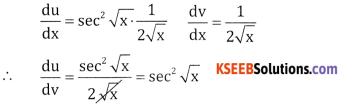Question 5.
Differentiate log x w.r.t $$\frac { 1 }{ x }$$
Let u = log x, v = $$\frac { 1 }{ x }$$Question 6.
Differentiate log sin x w.r.t $$\sqrt{\cos x}$$
Let u = log (sin x) v = $$\sqrt{\cos x}$$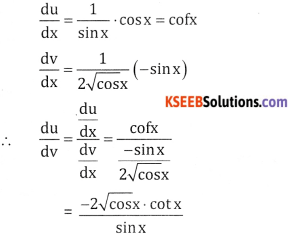Question 7.
If x = elog cos 4θ , y = elog sin 4θ show that $$\frac{d y}{d x}=\frac{-x}{y}$$
Given x = cos 4θ, y = sin 4θ
$$\frac{d x}{d \theta}$$ = -4sin 4θ
$$\frac{d y}{d \theta}$$ = + 4cos 4θ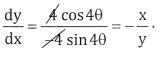Question 8.
If x = a cos4θ, y = asin 4θ show that $$\frac{d y}{d x}$$ = -tan2θ
Given x = a cos4θ, y = a sin 4θ
$$\frac{d x}{d \theta}$$ = a(4cos3θ( – sinθ),) $$\frac{d y}{d \theta}$$ = 4a sin3θ cos θ
∴ $$\frac{d y}{d x}=\frac{4 a \sin ^{3} \theta \cos \theta}{-4 a \cos ^{3} \theta \sin \theta}=-\tan ^{2} \theta$$

Question 9.
If x = et(cos t + sin t), y = et(cos t – sin t). show that $$\frac{d y}{d x}$$ = -tan t.If x = a log sec θ, y = a(tanθ – 1) show that $$\frac{d y}{d x}$$ = 2 cosec2θ